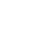# Use One Function Argument at a Time with Currying and Partial Application in PureScript

Published 4 years ago
Updated 3 years ago

Currying is a significant aspect of functional programming. It allows you to break down one function that takes many arguments into a series of functions that take part of the arguments; this will enable you to build and compose functions together easily.

In this lesson, we will look at what currying is doing under the hood in PureScript, as it is used extensively.

Join in by going to the online PureScript editor.

Instructor: [00:00] We'll create a function called addMe with the types of int to int to int, so addMe expects the values of a and b. Then, that = a+b. You might have been thinking, "What is the right arrow in a type definition?" It's the right associativity from one type to the other. Whoa, whoa, whoa, OK. We'll take a few steps back, and we'll explain exactly what this means.

[00:22] We'll create some pseudocode, and I'll put in some braces. Firstly, we have the outermost function, which is a. This goes into a function inside of it which is b. Inside of that, we have an a+b. I'll break it down a little more. The function a actually returns us a function which expects a b. Within that function, there is an a+b.

[00:45] At this point, it might still be a little bit confusing, so what we'll do is create a new function to further demonstrate what is going. At the same time, you'll get further understanding of currying. You could say that's two birds with one stone. Obviously, I don't condone that. Let's create a function called add5. Its signature will be int to int. Now on the next line, we'll do add5 = addMe 5.

[01:11] This is odd. The function addMe, and I've just put a value of 5. Where's its second value? First, let's see it in action, add5 with the value of 5. If you look to the right, that returns us 10. There might still be some confusion at this stage, so let's give add5 some more pseudocode. add5 only expects an int and returns an int. That's because we've partially applied a 5 to addMe.

[01:37] Let's remove this part of the pseudocode to show that, so add5 expects one value. If we look below, we pass in 5. In our pseudocode, that's the b. This is then fed into addMe. We'd partially applied our a value, and now we've given it our b. Now, addMe has all of the values a and b, which is 5+5.

[01:57] Let's write this slightly differently. We'll hide our a and b. I have a function which expects an a, and that returns a function which expects a b. That returns a function which has a+b. In add5, we call addMe and give it the a. This returns us a function that expects a b. When we're calling add5, we're giving it the second value. At that point, that returns us a+b, in our case, which is 5+5.

[02:28] The great trick to currying is you can pass it one value, and then, it returns you a function which is just expecting the second value. If you look at a type definition of addMe, it expects an int, which is our a. That returns us a function which expects another int, which is our b. At that point, we both have a and b, and we can return the final int, which is a calculation of a+b.

[02:51] You can see how we're using addMe and giving it just one of its inputs. Then, we're using add5 to give addMe its second input, which partially applying functions and chaining them together to give us a result. Doing that is called "currying."Erkan Buelbuel
~ 3 years ago

I see, World domination of the TypeScript is guaranteed. :)